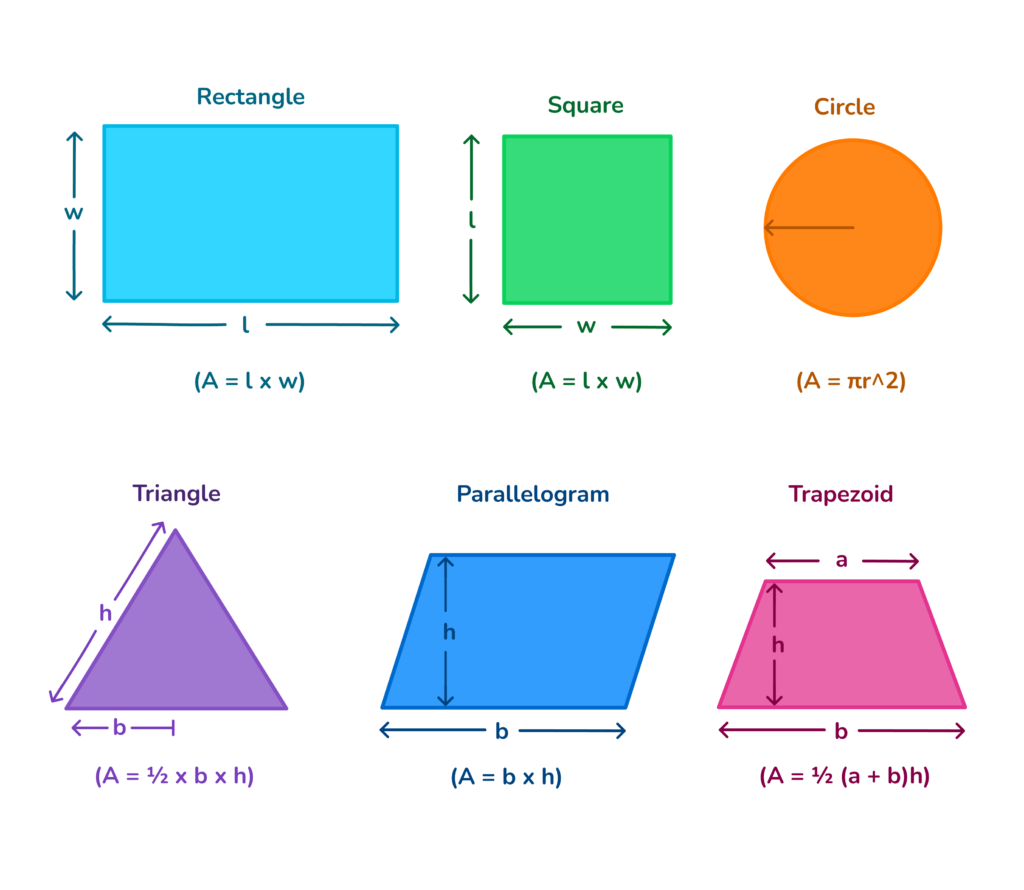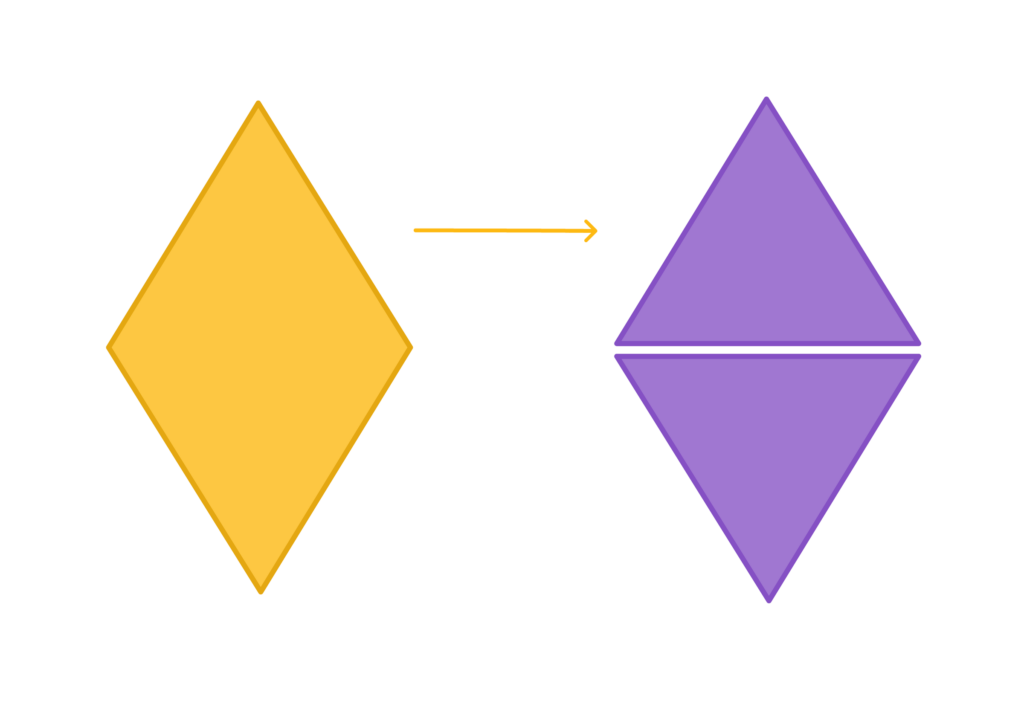# What is Perimeter in Math?

Perimeter is the measurement of length around a shape. You can think of it as a boundary or fence around your “geometric” yard.Author
Michelle GriczikaPublished
Nov 14, 2023# What is Perimeter in Math?

Perimeter is the measurement of length around a shape. You can think of it as a boundary or fence around your “geometric” yard.Author
Michelle GriczikaPublished
Nov 14, 2023# What is Perimeter in Math?

Perimeter is the measurement of length around a shape. You can think of it as a boundary or fence around your “geometric” yard.Author
Michelle GriczikaPublished
Nov 14, 2023Key takeaways

• Perimeter is the whole distance around a shape.
• Different shapes have unique formulas for perimeter.
• Finding the perimeter has many uses in everyday life like determining how much fence you need for your yard.

When you think of the outline of a shape or the boundary that encloses its space, you’re thinking of the perimeter. It’s the total distance around the edges of a geometric figure. This concept is crucial in math for helping us measure and compare different shapes.

## Perimeter DefinitionPerimeter refers to the total distance around a shape. It’s like tracing the edges or boundaries of a figure to measure its full length. In simpler terms, imagine tying a string around a shape; the length of that string represents the perimeter.

## Real-life examples of calculating perimeterThere are many uses for finding perimeter in everyday life. Calculating perimeter is especially important in construction or sports like distance running. Here are some real life scenarios where you may need to know the perimeter:

1. Fencing a Yard: If you want to fence your yard, you’d need to measure the total distance around it to buy the right amount of fencing. That distance is the perimeter!

2. Picture Frames: When choosing or making a frame for a painting or photograph, the frame’s outer edge or boundary length represents its perimeter.

3. Running Tracks: Athletes running on a track cover the perimeter of the shape. If they want to know how far they have to run, that’s the perimeter!## What is the formula for perimeter?Understanding perimeter involves knowing how to measure the distance around different shapes. Each shape has its unique formula to find the perimeter. Let’s look at a few of the common shapes and their formulas:

• Square or Rectangle: To calculate the perimeter of a square or rectangle, add up all its sides. For a square, where all sides are equal, you simply multiply one side by 4 (P = 4s). In a rectangle, you’ll add up the two longer sides and the two shorter sides, or double the length and add it to double the width (P = 2l + 2w).

• Circle: To measure the distance around a circle, or its perimeter, you use the perimeter formula two times pi times the radius (P = 2πr). Remember, the radius is the straight line from the circle’s center to its edge.

• Triangle: The perimeter of a triangle is the total of all its three sides. Just add side ‘a’ to side ‘b’ to side ‘c’, and there you have it (P = a + b +c)!

• Parallelogram: The perimeter of a parallelogram is found by adding up the lengths of all its sides. If the opposite sides are equal, then you can double one side and add it to double the other side (P = 2(a + b)).

• Trapezoid: For a trapezoid, you simply add all its four sides together to get it’s perimeter (P = a + b + c + d).Here’s a handy table with all the perimeter formulas for a quick look:

 Shape Formula for Perimeter Square P = 4s (where s = length of one side) Rectangle P = 2(l + w) or P = 2l + 2w Circle P = 2πr Triangle P = a + b + c Parallelogram P = 2(a + b) or P = 2a + 2b Trapezoid P = a + b + c + d

Remember, knowing the formula is just the start. Applying it practically helps strengthen your understanding. Dive deeper with our math app for more practice on perimeter calculations.

## How to find perimeterHow do you find perimeter? To find out how long the outside of a shape is, follow these steps:

### Finding the Perimeter of Regular Shapes

These are easy shapes like squares, circles, and rectangles.

Here’s what to do:

• Square: All four sides are the same. So, take the length of one side and multiply by 4.
• Circle: Use the formula P=2πr where π=3.14 and r=the length of the center of the circle to the edge.
• Rectangle: Add the top and bottom lengths, then the left and right lengths. Or, find the the length of the top of the rectangle and multiply by two and add that to the length of the side times two. Ex: 2L + 2W or L + L + W + W where L=length and W=width.

### Finding the Perimeter of Odd Shapes

For trickier shapes, try this:

1. Break it Down: Split the weird shape into easier ones like triangles or squares.
2. Measure Each Part: Find the outside length of these smaller shapes using the right formula.
3. Add Them All Up: Put together the lengths of these small shapes to get the total outside length.

In short, figure out the shape, use the right math, and you’ll get your answer. And remember, the formula table is super helpful!## Practice Problems for Finding PerimeterClick on the boxes below to see the answers!

Rectangle: 36 units

Square: 32 units

Triangle: 18 units

Circle: 25.12 units

Parallelogram: 30 units

Trapezoid: 34 units

Rectangle: 44 units

Square: 24 units

Triangle: 30 units

Circle: 31.4 units

## FAQs About Perimeter in MathPerimeter refers to the total distance around a shape. It measures the boundaries or edges of a figure.

To find the perimeter, you add up the lengths of all the sides of the shape. For certain regular shapes like rectangles or circles, specific formulas can be applied.

The perimeter of a rectangle is found by adding up all its sides, which includes twice the length and twice the width or 2(l + w).

Area measures the total space inside a shape, while perimeter measures the total distance around it.Title 1

Title 2

Title 3

## Related Posts

Title 1Title 2Title 3### In summary...Understanding area is a crucial part of math education. To further simplify the process, consider using resources like our app for math help. It’s an effective tool to assist learners in understanding these fundamental concepts through interactive lessons and practice problems.

Lesson credits

Michelle Griczika

Michelle Griczika is a seasoned educator and experienced freelance writer. Her years teaching first and fifth grades coupled with her double certification in elementary and early childhood education lend depth to her understanding of diverse learning stages. Michelle enjoys running in her free time and undertaking home projects.

Michelle Griczika

Michelle Griczika is a seasoned educator and experienced freelance writer. Her years teaching first and fifth grades coupled with her double certification in elementary and early childhood education lend depth to her understanding of diverse learning stages. Michelle enjoys running in her free time and undertaking home projects.# Are you a parent, teacher or student?

Are you a parent or teacher?

## Hi there!

Book a chat with our team